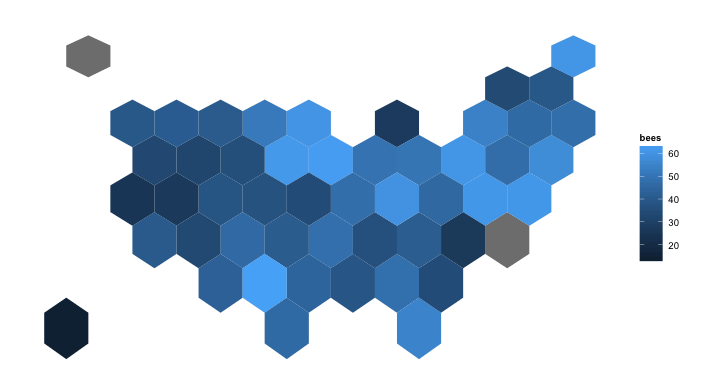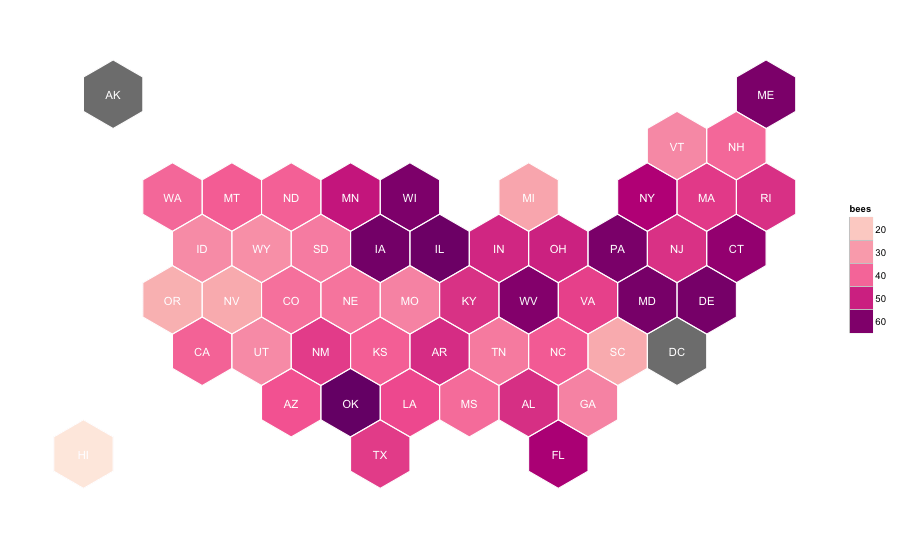# GeoJSON Hexagonal “Statebins” in R

There’s been lots of buzz about “statebin” maps of late. A recent tweet by @andrewxhill referencing work by @dannydb pointed to a nice shapefile (alternate link) that ends up being a really great way to handle statebin maps (and I feel like a fool for not considering it for a more generic solution earlier).

Here’s a way to use the GeoJSON version in R. I like GeoJSON since it’s a single file vs a directory of files and is readable vs binary. If you’re in a TL;DR hurry, you can just review the code in this gist. Read on for expository.

#### Taking a look around

When you download the GeoJSON, it should be in a file called `us_states_hexgrid.geojson`. We can see what’s in there with R pretty easily:

``````library(rgdal)

ogrInfo("us_states_hexgrid.geojson", "OGRGeoJSON")

## Source: "us_states_hexgrid.geojson", layer: "OGRGeoJSON"
## Driver: GeoJSON number of rows 51
## Feature type: wkbPolygon with 2 dimensions
## Extent: (-137.9747 26.39343) - (-69.90286 55.3132)
## CRS: +proj=longlat +datum=WGS84 +no_defs
## Number of fields: 6
##         name type length typeName
## 1 cartodb_id    0      0  Integer
## 2 created_at    4      0   String
## 3 updated_at    4      0   String
## 4      label    4      0   String
## 5       bees    2      0     Real
## 6  iso3166_2    4      0   String``````

Along with the basic shapefile goodness, we have some data, too! We’ll use all this to make a chorpleth hexbin of “bees” (I have no idea what this is but assume it has something to do with bee population, which is a serious problem on the planet right now). Let’s dig in.

#### Plotting the bins

First we need to read in the data, which is pretty simple:

``````

That ends up being a fairly complex object with polygons and data. However, we can take a quick look at it with base R graphics:

``````
plot(us)``````Yay! While we could do most (if not all) the remainder of the graphics in base R, I personally believe ggplot is more intuitive and expressive, so let's do the same thing with ggplot. First, we'll have to get the data structure into something ggplot can handle:

``````library(ggplot2)

us_map <- fortify(us, region="iso3166_2")``````

That gives us a data frame with the 2-letter state abbreviations as the "region" keys. Now we can do a basic ggplot:

``````ggplot(data=us_map, aes(map_id=id, x=long, y=lat)) +
geom_map(map=us_map, color="black", fill="white")``````Ugh. Talk about ugly. But, at least it works! Now all we need to do is turn it into a choropleth, remove some map chart junk and make it look prettier!

#### Upping the aesthetics

There's a pretty good idiom for making maps in R. There's a handy layer/`geom` called `geom_map` which takes care of a ton of details under the hood. We can use it for making outlines and fills and add as many layers of them as we want/need. For our needs, we'll:

• put down a base layer of polygons
• add a fill layer for our data
• get rid of map chart junk

This is all pretty straightforward once you get the hang of it:

``````g <- ggplot()

# Plot base map -----------------------------------------------------------

gg <- gg + geom_map(data=us_map, map=us_map,
aes(x=long, y=lat, map_id=id),
color="white", size=0.5)

# Plot filled polygons ----------------------------------------------------

gg <- gg + geom_map(data=us@data, map=us_map,
aes(fill=bees, map_id=iso3166_2))

# Remove chart junk for the “map" -----------------------------------------

gg <- gg + labs(x=NULL, y=NULL)
gg <- gg + theme_bw()
gg <- gg + theme(panel.border=element_blank())
gg <- gg + theme(panel.grid=element_blank())
gg <- gg + theme(axis.ticks=element_blank())
gg <- gg + theme(axis.text=element_blank())
gg``````Definitely better, but it still needs work. Outlines would be good and it definitely needs a better color palette. It would also be nice if the polygons weren't "warped". We can fix these issues by adding in a few other elements:

``````gg <- ggplot()
gg <- gg + geom_map(data=us_map, map=us_map,
aes(x=long, y=lat, map_id=id),
color="white", size=0.5)
gg <- gg + geom_map(data=us@data, map=us_map,
aes(fill=bees, map_id=iso3166_2))

# Overlay borders without ugly line on legend -----------------------------

gg <- gg + geom_map(data=us@data, map=us_map,
aes(map_id=iso3166_2),
fill="#ffffff", alpha=0, color="white",
show_guide=FALSE)

# ColorBrewer scale; using distiller for discrete vs continuous -----------

gg <- gg + scale_fill_distiller(palette="RdPu", na.value="#7f7f7f")

# coord_map mercator works best for the display ---------------------------

gg <- gg + coord_map()

gg <- gg + labs(x=NULL, y=NULL)
gg <- gg + theme_bw()
gg <- gg + theme(panel.border=element_blank())
gg <- gg + theme(panel.grid=element_blank())
gg <- gg + theme(axis.ticks=element_blank())
gg <- gg + theme(axis.text=element_blank())
gg``````Much better. We use a "hack" to keep the legend free of white slash marks for the polygon outlines (see the comments for a less-hackish way) and `coord_map` to let the projection handle the "unwarping". By using the `distiller` fill, we get discrete color bins vs continuous shades (use what you feel is most appropriate, though, for your own work).

#### Where are we?

Most statebin/hexbin maps still (probably) need state labels since (a) Americans are notoriously bad at geography and (b) even if they were good at geography, we've removed much of the base references for folks to work from accurately.

The data exists in the shapefile, but to get the labels put in the centers of each polygon we have to do a bit of work:

``````library(rgeos)

centers <- cbind.data.frame(data.frame(gCentroid(us, byid=TRUE), id=us@data\$iso3166_2))``````

That gets us a data frame of the `x` & `y` centers of each polygon along with the (abbreviated) state name. We can now add a layer with `geom_text` to place the label. The following is the complete solution:

``````library(rgdal)
library(rgeos)
library(ggplot2)

centers <- cbind.data.frame(data.frame(gCentroid(us, byid=TRUE), id=us@data\$iso3166_2))

us_map <- fortify(us, region="iso3166_2")

ggplot(data=us_map, aes(map_id=id, x=long, y=lat)) + geom_map(map=us_map, color="black", fill="white")

gg <- ggplot()
gg <- gg + geom_map(data=us_map, map=us_map,
aes(x=long, y=lat, map_id=id),
color="white", size=0.5)
gg <- gg + geom_map(data=us@data, map=us_map,
aes(fill=bees, map_id=iso3166_2))
gg <- gg + geom_map(data=us@data, map=us_map,
aes(map_id=iso3166_2),
fill="#ffffff", alpha=0, color="white",
show_guide=FALSE)
gg <- gg + geom_text(data=centers, aes(label=id, x=x, y=y), color="white", size=4)
gg <- gg + scale_fill_distiller(palette="RdPu", na.value="#7f7f7f")
gg <- gg + coord_map()
gg <- gg + labs(x=NULL, y=NULL)
gg <- gg + theme_bw()
gg <- gg + theme(panel.border=element_blank())
gg <- gg + theme(panel.grid=element_blank())
gg <- gg + theme(axis.ticks=element_blank())
gg <- gg + theme(axis.text=element_blank())
gg``````#### Wrapping up

This is a pretty neat way to work with "statebins" and I'll probably take some time over the summer to update my statebins package to use shapefiles and allow for more generic shapes. Ramnath Vaidyanathan has also done some work with statebins and javascript, so I'll see what I can do to merge all the functionality into one package.

If you've got an alternate way to work with these or have some interesting "bins" to show, drop a note in the comments.Amazon Author Page

## 10 Comments →GeoJSON Hexagonal “Statebins” in R

1. Pingback: GeoJSON Hexagonal “Statebins” in R | infopunk.org

2.Gregor Thomas

This is really nice! A less hack-ish way to get rid of the white slash in the legend is `guides(fill = guide_legend(override.aes = list(colour = NA)))`

3.akm

Why are Alaska and DC the same out-of-palette colors?

1.hrbrmstr

Good q! If you look at the data you’ll see that there are no values for them, so they get assigned the `na.value` color.

4.SaS

Great job ! Thank you very much for sharing. Do you have any idea where to find an hexagonal-bin world map ?

5.shelloj

Is there anyway to get Puerto Rico in to this type of map?

1.hrbrmstr

The original GeoJSON file is fairly minimal. It wouldn’t take much to add a hex for PR. FWIW my `statebins` package has PR in it. No hexes but lovely squares.

1.SHELLOJ

Thanks Bob! Appreciate all the code you’ve shared :)

This site uses Akismet to reduce spam. Learn how your comment data is processed.